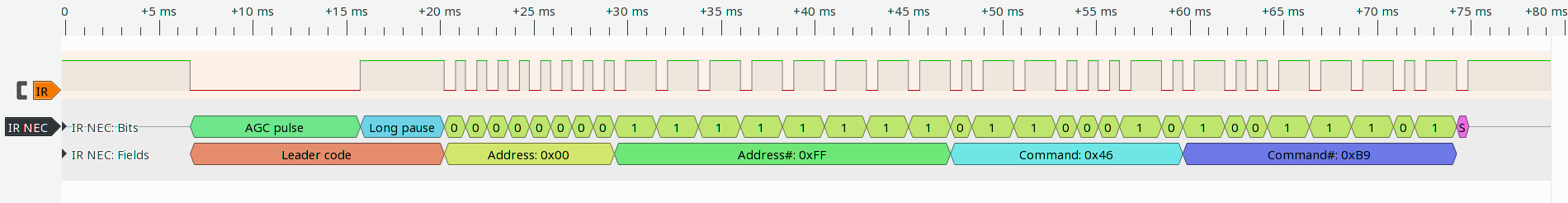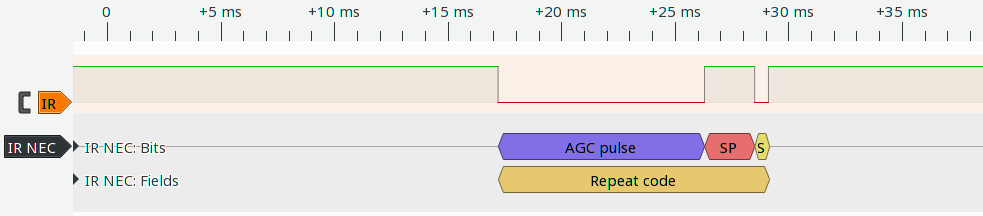Ever wondered how IR remote controls work? Let's look into it and use one to control an LED connected to our Arduino.

## IR Remote Controls

A simple way to transmit data using light is to use Morse code. Maybe you tried it using a flashlight, once you were a kid. IR remote controls work in a quite similar fashion. There is a problem, however. The sun light contains infrared light as well, and you don't want your TV to turn on due to sun light. To solve this a so-called carrier frequency is used. Typically, the pulses are transmitted as a 38 kHz signal. The IR receiver will only react to infrared signals at this frequency. To transmit data the 38 kHz signal is turned on and off. The receiver will transform this into a digital signal. In case of the receiver I used a low voltage level is output if the 38 kHz carrier signal is detected.

Let's take look at the output of the IR receiver:The start of the transmission is indicated by a long pulse. This start pulse is followed by 32 data bits and a final stop pulse. For a zero bit there is only a short gap in the infrared signal and for a one there is a longer gap. These data bits vary for each key on the remote and also for remotes of different devices. The protocol used by most of the Arduino IR remotes is the NEC protocol. There are other protocols and even other carrier frequencies as well, but the NEC protocol is pretty common and used by all my TV remotes.

The NEC protocol includes a key repeat package as well, with includes no data, but instead signals that the key is still pressed. The corresponding data package is shown below:The goal of this tutorial is to turn an LED on and off using the remote and our Arduino Uno. For this purpose we will use one key of the remote to turn the LED on and another one to turn it off. In our use case we can just ignore all key repeat packages.

## Building the CircuitFor our circuit we need the LED which we want to control and an IR receiver. The LED is connected as usual with a 220 Ω resistors in series. I used pin 9 to control the LED. The IR receiver has three connections. One of them is the data line which I connected to pin 8. The other two pins are used to supply power. One is connected to 5V and the other to 0V. I used a VS1838B receiver. Other receivers that match the carrier frequency and wavelength of the remote will work as well. The pin assignment might differ, however. In the video at the end of this tutorial you will see that the pin assignment of my receiver differs from the one shown in the wiring diagram above. My receiver faces in the opposite direction. Please make sure to adjust the wiring to your receiver.

## Writing the Code

Our final goal is to control the LED using the remote. To start, we should divide this task into smaller steps. At first, we need a way to measure the length of the pulses. We need to transform the pulse lengths into a value, which we can later use to determine which key got pressed. Once we are able to differentiate between the individual keys of the remote we can use two of them to switch the LED on and off.

### Measuring the Pulse Lengths

In the introduction to IR remotes I showed you how the transmitted signal looks like. We can determine if a bit is zero or one by measuring the length of the gap between pulses. During this gap pin 8 is HIGH for a short period of time. The Arduino platform provides the function pulseIn for measuring the length of this HIGH pulse at pin 8. Here is the description of this function:

• pulseIn(pin, value, timeout)
• pin: Number of the pin to measure the pulse length
• value: HIGH or LOW depending on whether we want to measure the length of periods with low or high voltage level
• timeout: Optional parameter, to specify the maximum expected pulse length in microseconds (1 s if omitted)
• Returns the pulse length in microseconds or 0 if the timeout is exceeded

To test the function and get an impression of what pulse lengths we have to expect we can just log the pulse lengths to the serial console. We need to adjust the baud rate to a higher value than usual, because otherwise the transmission to the computer takes too long and we miss the next pulse. A baud rate of 115200 worked for me. Remember to choose the same baud rate in the serial monitor.

The following code logs the length of each HIGH pulse in the serial monitor:

void setup() {
Serial.begin(115200);
pinMode(8, INPUT);
}

void loop() {
Serial.println(pulseIn(8, HIGH));
}

### Decoding the Data

The more difficult part is to decode the measured pulse lengths and produce a value from it that we can use to identify the pressed key. As we have 32 data bits transmitted by the remote it makes sense to construct a 32-bit integer from them. But how can we do this?

Let me show you the code first and then explain how it works.

void setup() {
Serial.begin(9600);
pinMode(8, INPUT);
}

// Wait for start pulse
if(pulseIn(8, HIGH, 20000) == 0) return 0;

unsigned long int result = 0;
for(int i = 0; i < 32; i++) {
int pulseLength = pulseIn(8, HIGH, 5000);
if(pulseLength == 0) return 0;
if(pulseLength > 1000) result |= (1UL<<i);
}

// Stop pulse

return result;
}

void loop() {
}

I declared a new function to receive and decode the IR signal. In loop I just send its return value as a hexadecimal number to the serial console. We have not used the second parameter of Serial.println before, but there is not much more to say than the fact that it changes the display format for integer numbers. Possible values are BIN, OCT, DEC and HEX for displaying numbers in binary, octal, decimal or hexadecimal notation.

The magic happens inside the receiveIR function. First we wait for the start pulse to arrive and for the pause right after it. This gap is about 10 ms long, so that a timeout of 20 ms should be enough. If we don't get this gap we abort. Maybe the remote was out of range or uses an unknown protocol. Next we read the 32 data bits inside the for loop. To do so, we measure the gap length using the pulseIn function. In the previous step we measured the length of these gaps. With my remote there is a short gap of 500 µs for a zero bit and a longer gap of 1500 µs for a one. I therefore choose a timeout of 5000 µs for measuring the pulse length. This value should be never exceeded for data bits. If it is exceeded this may be because of a stop bit, as in the key repeat package, or because of a signal interruption. In both cases we won't be able to read data. The timeout will exceed andpulseIn will return 0. In this case the receiveIR functions aborts by just returning 0 to indicate that no data was read.

For decoding the input signal, we need to combine the received bits to our 32-bit integer result. To set a bit in an integer we can use a bitwise OR. In C++ the | operator is used for this. The operator |= is just a shortcut for result = result | (1UL << 1). In the result of a bitwise OR all bits that have a value of one in one of the two integers is set to one. In the beginning, all bits are zero because we initialized result with 0. Each time we measure a gap longer than 1000 µs, we have received a one. In this case we need to set the corresponding bit in result to one using the bitwise OR. The bits are transferred with the least significant bit first. The first data bit we receive is bit 0 in our integer. This is followed by bit 1, then bit 2 and so on. In case of a one for bit 3 we would need to execute result |= 8 to set bit 3, as 8 is the number with bit 3 set to one.

The more generic way to do this, is using a shift operator.
The << operator shifts all bits in an integer to the left:

A value of 1 represented as bits ...

Bit 7 6 5 4 3 2 1 0
State 0 0 0 0 1 0 0 0
Value 1

... shifted by 3 to the left (1<<3), results in a value of 8

Bit 7 6 5 4 3 2 1 0
State 0 0 0 0 1 0 0 0
Value 8

In the loop we use the variable i to iterate over all bits from 0 to 31 and set them to one, if we receive a one from the IR remote control. This is achieved by shifting 1 to the left by i and then setting the ith bit using the bitwise OR. As a result we get a number that can be used to uniquely identify the pressed key. One last thing that might be a bit confusing is the fact that 1UL is used instead of 1. This explicitly states that this 1 is of the type unsigned long. It is needed because the compiler would assume a normal 16-bit integer otherwise. As a result we would always get 0, if we shift by more than 15 bits, as bits shifted out on the left end just get dropped.

To finish, the stop pulse. We just wait until the data line returns to a HIGH state. The decoded key code in result is then returned by the function.

### Controlling the LED

To control the LED using the remote, we need to pick two keys. By using a condition we can check if one of these keys was pressed and set the LED to the corresponding state using digitalWrite. To find the key codes, we can use the program we just wrote and look up the codes in the serial monitor. You might need to adjust the key code values to your remote.

Here is the complete code, which uses two keys of my remote (CH- and CH+) to turn the LED on and off:

void setup() {
pinMode(8, INPUT);
pinMode(9, OUTPUT);
}

// Wait for start pulse
if(pulseIn(8, HIGH, 20000) == 0) return 0;

unsigned long int result = 0;
for(int i = 0; i < 32; i++) {
int pulseLength = pulseIn(8, HIGH, 5000);
if(pulseLength == 0) return 0;
if(pulseLength > 1000) result |= (1UL<<i);
}

// Stop pulse

return result;
}

void loop() {
}